• Last Updated : 13 Aug, 2020

These are following important points about circle in geometry :

1. Equation of circle having center at (0, 0) and radius r :
`x2+y2=r2 `
2. Equation of circle having center at (h, k) and radius a :
`(x-h)2+(y-k)2=a2 `
3. Standard equation of circle is x2+y2+2gx+2fy+c=0 where radius=?(g2+f2-c) and center at(-g, -f) and condition is g2+f2-c > = 0
4. If  g2+f2-c=0 then equation represents a point circle having center only (-g, -f).
5. Diametrical form of a circle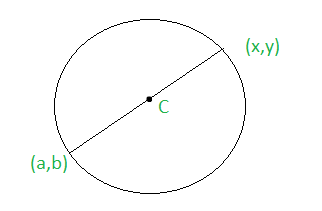Figure – (X-x)(X-a)+(Y-y)(Y-b) = 0

```S1=x12+y12+2gx1+2fy1+c
S2=x22+y22+2gx2+2fy2+c
```
6. Equation of Circle Passing through point of intersection of circles S1=0 and S2=0 is S1+kS2=0 where k is not equal to -1.
7. Equation of circle passing through a point of intersection of circle s=0 and line u=0 is s+ku=0
8. If the circles S1=0 and S2=0 intersect then S1-S2=0 is their common chord.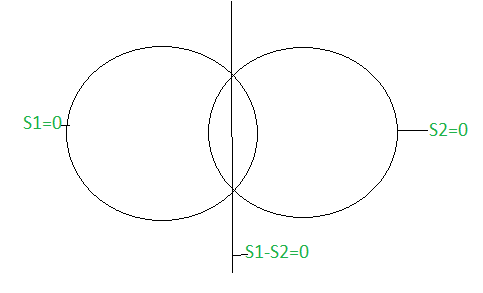9. If two circles S1=0 and S2=0 have internal contact the S1-S2=0 is their internal common tangent.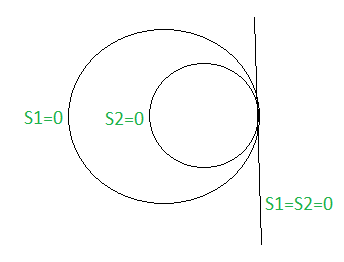10. If Two Circles S1=0 and S2=0 do not intersect then S1-S2=0 is their radial axis.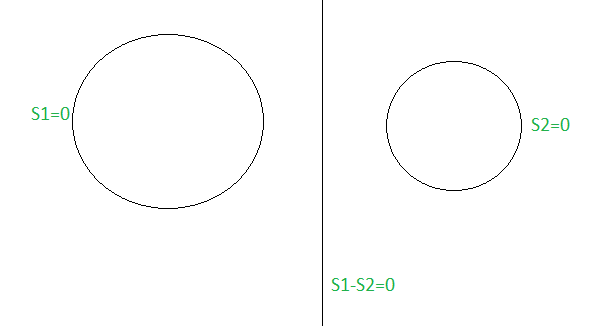11. If Two Circles S1=0 and S2=0 have external contact the S1-S2=0 is their external common tangent.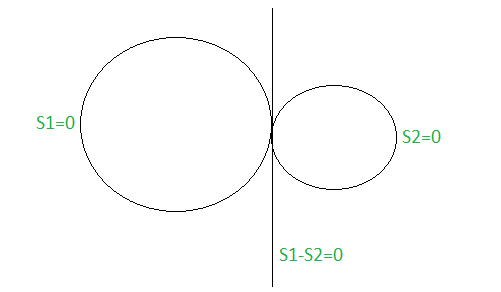My Personal Notes arrow_drop_up
Recommended Articles
Page :